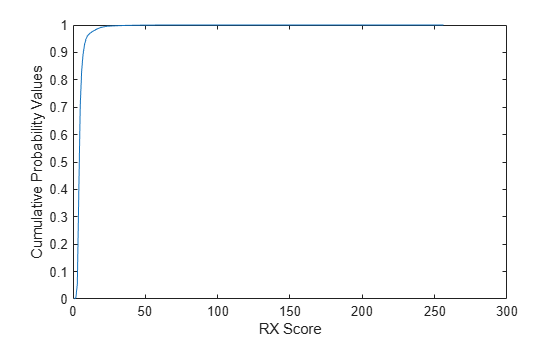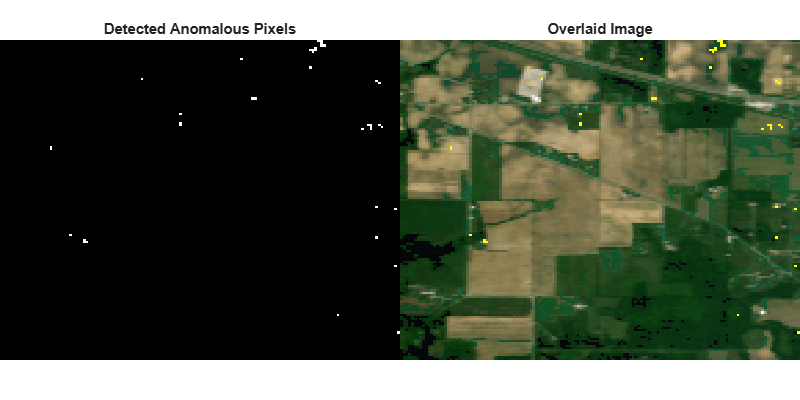# anomalyRX

Detect anomalies using Reed-Xiaoli detector

## Syntax

``rxScore = anomalyRX(inputData)``

## Description

example

````rxScore = anomalyRX(inputData)` detects anomalous pixels in the hyperspectral data using the Reed-Xialoi (RX) detector. The RX detector calculates a score for each pixel as the Mahalanobis distance between the pixel and the background. The higher score indicates a likely anomaly. The background is characterized by the spectral mean and covariance of the data cube. For more information about computing the score and detecting anomalies, see Algorithms. NoteThis function requires the Image Processing Toolbox™ Hyperspectral Imaging Library. You can install the Image Processing Toolbox Hyperspectral Imaging Library from Add-On Explorer. For more information about installing add-ons, see Get and Manage Add-Ons. ```

## Examples

collapse all

Detect anomalous pixels in hyperspectral data by computing the RX score for each pixel in a hyperspectral data cube. Then compute the threshold for detecting true anomalous pixels by using cumulative probability distribution of RX score values.

Read hyperspectral data containing anomalous pixels into the workspace.

`hcube = hypercube('indian_pines.dat');`

Find anomalous pixels in the input hyperspectral data by using the RX detector. The detector searches for pixels with a high intensity difference within a homogeneous region.

`rxScore = anomalyRX(hcube);`

Reduce the dynamic range of the RX score values by rescaling them to the range [0, 255].

`rxScore = im2uint8(rescale(rxScore));`

Display the RX score map. Pixels with a high RX score are likely anomalous pixels.

```figure imagesc(rxScore) colorbar```Compute and plot the cumulative probability distribution of RX score values.

```count = imhist(rxScore); pdf = count/prod(size(rxScore,[1 2])); cdf = cumsum(pdf(:)); figure plot(cdf) xlabel('RX Score') ylabel('Cumulative Probability Values')```Set the confidence coefficient value to 0.998. Select the first RX score with cumulative probability distribution value greater than the confidence coefficient as the threshold. This threshold represents the RX score above which a pixel is an anomaly with 99.8 percent confidence.

```confCoefficient = 0.998; rxThreshold = find(cdf > confCoefficient,1);```

Apply thresholding to detect anomalous pixels with RX score greater than the computed threshold. The result is a binary image in which the anomalous pixels are assigned the intensity value 1 and other pixels are assigned 0.

`bw = rxScore > rxThreshold;`

Derive the RGB version of the data cube by using the `colorize` function. Overlay the binary image of anomalous pixels on the RGB image.

```rgbImg = colorize(hcube,'Method','rgb'); B = imoverlay(rgbImg,bw);```

Display both the binary image and the overlaid image.

```fig = figure('Position',[0 0 800 400]); axes1 = axes('Parent',fig,'Position',[0 0.1 0.5 0.8]); imagesc(bw,'Parent',axes1); title('Detected Anomalous Pixels') axis off colormap gray axes2 = axes('Parent',fig,'Position',[0.5 0.1 0.5 0.8]); imagesc(B,'Parent',axes2) title('Overlaid Image'); axis off ```## Input Arguments

collapse all

Input hyperspectral data, specified as a 3-D numeric array or `hypercube` object. If the input is an 3-D numeric array of size M-by-N-by-C, the function reads it as a hyperspectral data cube of M-by-N pixels with C spectral bands and computes the RX score. If the input is a `hypercube` object, the function reads the data cube stored in the `DataCube` property and then computes the RX score. The hyperspectral data cube must be real and non-sparse.

Data Types: `single` | `double` | `int8` | `int16` | `int32` | `int64` | `uint8` | `uint16` | `uint32` | `uint64`

## Output Arguments

collapse all

Output RX score for each pixel in the hyperspectral data cube, returned as a matrix of size M-by-N, same as the spatial dimensions of the input data.

Data Types: `double`

## Algorithms

The RX score for each pixel is computed as

`${D}_{RX}={\left(r-{\mu }_{C}\right)}^{T}{\Sigma }_{C}^{-1}\left(r-{\mu }_{C}\right)$`

r is the pixel under test and μC and ΣC are the spectral mean and covariance respectively. Anomalous pixels typically have the high RX scores.

You can estimate a threshold from the cumulative probability distribution of the RX scores to further tune the anomalous pixel detection. See the Detect Anomalous Pixels in Hyperspectral Data Using RX Detector example.

 Reed, I.S., and X. Yu. “Adaptive Multiple-Band CFAR Detection of an Optical Pattern with Unknown Spectral Distribution.” IEEE Transactions on Acoustics, Speech, and Signal Processing 38, no. 10 (October 1990): 1760–70. https://doi.org/10.1109/29.60107.

 Chein-I Chang and Shao-Shan Chiang. “Anomaly Detection and Classification for Hyperspectral Imagery.” IEEE Transactions on Geoscience and Remote Sensing 40, no. 6 (June 2002): 1314–25. https://doi.org/10.1109/TGRS.2002.800280.